## Monomials

A monomial is an algebraic expression that consists of only one term. (A term is a numerical or literal expression with its own sign.) For instance, 9 x, 4 a2, and 3 mpx2 are all monomials. The number in front of the variable is called the numerical coefficient. In 9 x, 9 is the coefficient.

To add or subtract monomials, follow the same rules as with signed numbers, provided that the terms are alike. Notice that you add or subtract the coefficients only and leave the variables the same.

##### Example 1

Perform the operation indicated.

1.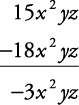2. 3 x + 2 x = 5 x

3.4.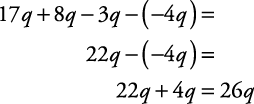Remember that the rules for signed numbers apply to monomials as well.

#### Multiplying monomials

Reminder: The rules and definitions for powers and exponents also apply in algebra.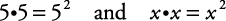Similarly, a · a · a · b · b = a3 b2.

To multiply monomials, add the exponents of the same bases.

##### Example 2

Multiply the following.

1. ( x3)( x4) = x3 + 4 = x7

2. ( x2 y)( x3 y2) = ( x2 x3)( yy2) = x2 + 3 y1 + 2 = x5 y3

3. (6 k5)(5 k2) = (6 × 5)( k5 k2) = 30 k5 + 2 = 30 k7 (multiply numbers)

4. –4( m2 n)(–3 m4 n3) = [(–4)(–3)]( m2 m4)( nn3) = 12 m2 + 4 n1 + 3 = 12 m6 n4 (multiply numbers)

5. ( c2)( c3)( c4) = c2 + 3 + 4 = c9

6. (3 a2 b3 c)( b2 c2 d) = 3( a2)( b3 b2)( cc2)( d) = 3 a2 b3 + 2 c1 + 2 d = 3 a2 b5 c3 d

Note that in example (d) the product of –4 and –3 is +12, the product of m2 and m4 is m6, and the product of n and n3 is n4, because any monomial having no exponent indicated is assumed to have an exponent of l.

When monomials are being raised to a power, the answer is obtained by multiplying the exponents of each part of the monomial by the power to which it is being raised.

##### Example 3

Simplify.

1. ( a7)3 = a21

2. ( x3 y2)4 = x12 y8

3. (2 x2 y3)3 = (2)3 x6 y9 = 8 x6 y9

#### Dividing monomials

To divide monomials, subtract the exponent of the divisor from the exponent of the dividend of the same base.

##### Example 4

Divide.

1.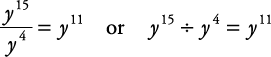2.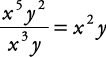3.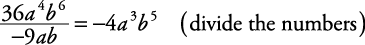4.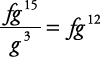5.6.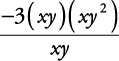You can simplify the numerator first.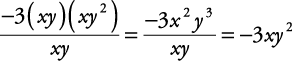Or, because is the numerator is all multiplication, you can reduce,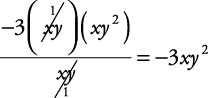#### Working with negative exponents

Remember, if the exponent is negative, such as x–3, then the variable and exponent may be dropped under the number 1 in a fraction to remove the negative sign as follows.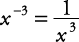##### Example 5

Express the answers with positive exponents.

1.2.3.